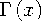Functions and CALL Routines

# GAMMA Function

Returns the value of the gamma function.
 Category: Mathematical

## Syntax

 GAMMA(argument)

### Arguments

argument

specifies a numeric constant, variable, or expression.

 Restriction: Nonpositive integers are invalid.

The GAMMA function returns the integral given byFor positive integers, GAMMA(x) is (x - 1)!. This function is commonly denoted by.

SAS Statements Results
`x=gamma(6);`
`120`Previous Page | Next Page | Top of Page